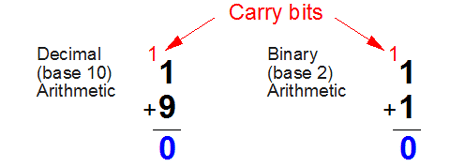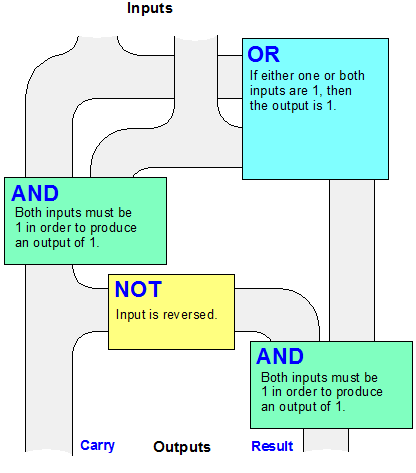# Definition: Boolean logic

The "mathematics of logic," developed by English mathematician George Boole in the mid-19th century. Just as add, subtract, multiply and divide are the primary operations of arithmetic, AND, OR and NOT are the primary logical operators of Boolean logic and building blocks of every digital circuit. NAND (not AND), NOR (not OR) and XOR (exclusive OR) are variations of AND, OR and NOT (see NAND, NOR and XOR). See Boolean search, binary, logic gate and Bebop to the Boolean Boogie.AND, OR and NOT Gates
Transistors wired in series and parallel patterns make up "gates," which accept binary input (0 = no pulse; 1 = pulse) and generate binary output. Although AND requires both inputs to be 1 in order to generate a 1 as output, OR only needs one. NOT reverses the input. For a diagram of these actions, see Boolean gates.Decimal vs. Binary
Decimal arithmetic holds 10 values (0 to 9) in each digit position. In binary, there are only two (0 and 1). Below are the four possible result and carry bits when adding two binary digits (bits) together.Trace the red 1 (pulse, current) and white 0 (no pulse, no current) through the gates and notice their outputs. This half-adder circuit is in every CPU.Trace the Flow Yourself
Choose any two binary digits as input and trace them through this half-adder circuit (0 is no current; 1 is current). Watch how the output is generated.Patterns of Boolean Logic
Transistors (depicted here as mechanical switches) make up gates. Gates make up circuits, and circuits make up every digital device. The most advanced chips have billions of transistors that all fit in an area smaller than a postage stamp. For more details, see Boolean gates. See transistor and chip.